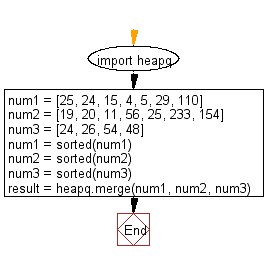﻿ Python heap queue algorithm: Merge multiple sorted inputs into a single sorted iterator using Heap queue algorithm - w3resource

# Python: Merge multiple sorted inputs into a single sorted iterator (over the sorted values) using Heap queue algorithm

## Python heap queue algorithm: Exercise-11 with Solution

Write a Python program that merges multiple sorted inputs into a single sorted iterator (over the sorted values) using the heap queue algorithm.

Sample Solution:

Python Code:

``````import heapq
num1 = [25, 24, 15, 4, 5, 29, 110]
num2 = [19, 20, 11, 56, 25, 233, 154]
num3 = [24, 26, 54, 48]
num1 = sorted(num1)
num2 = sorted(num2)
num3 = sorted(num3)
result = heapq.merge(num1, num2, num3)
print(list(result))
```
```

Sample Output:

```[4, 5, 11, 15, 19, 20, 24, 24, 25, 25, 26, 29, 48, 54, 56, 110, 154, 233]
```

Flowchart:Python Code Editor:

Have another way to solve this solution? Contribute your code (and comments) through Disqus.

What is the difficulty level of this exercise?

Test your Programming skills with w3resource's quiz.

﻿